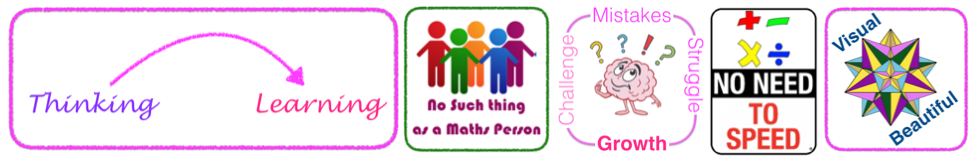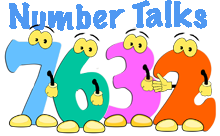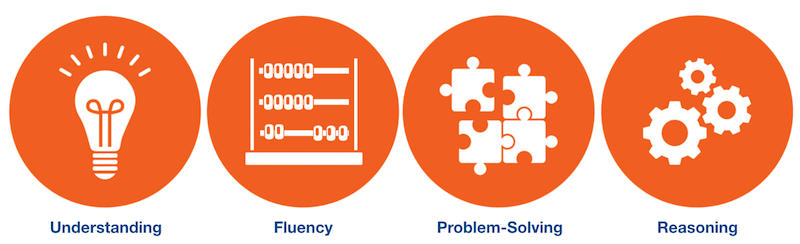# Working Mathematically

Working Mathematically relates to the syllabus objective:

Students develop understanding and fluency in mathematics through inquiry, exploring and connecting mathematical concepts, choosing and applying problem-solving skills and mathematical techniques, communication and reasoning

As an essential part of the learning process, Working Mathematically provides students with the opportunity to engage in genuine mathematical activity and develop the skills to become flexible and creative users of mathematics.

Working Mathematically is placed deliberately at the heart of the NESA Mathematics syllabus due to the central role it needs to play in K-10 teaching & learning.

A low-floor, high-ceiling task is one in which everyone can engage, no matter what his or her prior understanding or knowledge, but also one that is open enough to extend to high levels, so that all students can be deeply challenged.
Boaler, Munson & Williams (2018) p2

### Underlying Beliefs:• All Learning is a Consequence of Thinking - (HGSE Project Zero)
• There is no such thing as a math person— anyone can learn mathematics to high levels.
• Mistakes, struggle, and challenge are critical for brain growth.
• Speed is unimportant in mathematics.
• Mathematics is a visual and beautiful subject, and our brains want to think visually about mathematics.
Boaler, Munson & Williams (2018) p3

## In Mathematics, the key ideas are the proficiency strands of understanding, fluency, problem-solving and reasoning. The proficiency strands describe the actions in which students can engage when learning and using the content.

ACARA, Australian Mathematics Curriculum

### Communicating

Students develop the ability to use a variety of representations, in written, oral or graphical form, to formulate and express mathematical ideas. They are communicating mathematically when they describe, represent and explain mathematical situations, concepts, methods and solutions to problems, using appropriate language, terminology, tables, diagrams, graphs, symbols, notation and conventions.

### Problem Solving

Students develop the ability to make choices, interpret, formulate, model and investigate problem situations, and communicate solutions effectively. They formulate and solve problems when they use mathematics to represent unfamiliar or meaningful situations, design investigations and plan their approaches, apply strategies to seek solutions, and verify that their answers are reasonable.

### Reasoning

Students develop an increasingly sophisticated capacity for logical thought and actions, such as analysing, proving, evaluating, explaining, inferring, justifying and generalising. They are reasoning mathematically when they explain their thinking, deduce and justify strategies used and conclusions reached, adapt the known to the unknown, transfer learning from one context to another, prove that something is true or false, and compare and contrast related ideas and explain their choices.

But can you explain it?

"What is the point of my child explaining their work if they can get the answer right? My answer is always the same: Explaining your work is what, in mathematics, we call reasoning, and reasoning is central to the discipline of mathematics." Jo Boaler

The implication here from both the NESA Syllabus and from the position of research on effective pedagogies for Mathematics is clear; being able to attain a correct answer through the application of a learned strategy is not sufficient. Students must develop an understanding of the mathematics and this is demonstrated through their capacity to reason.

### Understanding*

Students build a strong foundation that enables them to adapt and transfer mathematical concepts. They make connections between related concepts and progressively apply the familiar to develop new ideas. Students develop an understanding of the relationship between the ‘why’ and the ‘how’ of mathematics. They build understanding when they connect related ideas, represent concepts in different ways, identify commonalities and differences between aspects of content, describe their thinking mathematically, and interpret mathematical information.

### Fluency*

Students develop skills in choosing appropriate procedures, carrying out procedures flexibly, accurately, efficiently and appropriately, and recalling factual knowledge and concepts readily. They are fluent when they calculate answers efficiently, recognise robust ways of answering questions, choose appropriate methods and approximations, recall definitions and regularly use facts, and manipulate expressions and equations to find solutions.

"The five components of Working Mathematically describe how content is explored or developed − that is, the thinking and doing of mathematics. They provide the language to build in the developmental aspects of the learning of mathematics. The components come into play when students are developing new skills and concepts, and also when they are applying their existing knowledge to solve routine and non-routine problems both within and beyond mathematics." NESA - p37Fluency is well supported through the use of Number Talks. - "Number talks are a valuable classroom routine for developing efficient computational strategies, making sense of math, and communicating mathematical reasoning. A number talk is structured to help students conceptually understand math without memorizing a set of rules and procedures." (Nancy Hughes)

### Fluency is Not Related to Speed

There is a common misconception that speed is a suitable measure of fluency, particularly when this relates to recall of multiplication facts. this is not the case and many talented mathematicians are slow thinkers. When students are asked to complete timed tests, engage in competitions where speed is required or miss opportunities to share their thinking because others are quicker, they develop a negative attitude to mathematics.

Laurent Schwartz won the Fields Medal in 1950 for his work on distributions. The Fields Medal is considered the Nobel prize of Mathematics and the highest honour a mathematician can receive. In his autobiography he shares his frustration with the false connection between speed, fluency and intelligence.

I was always deeply uncertain about my own intellectual capacity; I thought I was unintelligent. And it is true that I was, and still am, rather slow. I need time to seize things because I always need to understand them fully. Towards the end of the eleventh grade, I secretly thought of myself as stupid. I worried about this for a long time.

I'm still just as slow… At the end of the eleventh grade, I took the measure of the situation, and came to the conclusion that rapidity doesn't have a precise relation to intelligence. What is important is to deeply understand things and their relations to each other. This is where intelligence lies. The fact of being quick or slow isn't really relevant. (Schwartz, 2001)### The role of Representation in Mathematical Learning

At all stages of mathematical learning and in all areas of mathematics, students benefit from exploring concepts through multiple representations. In mathematics, representation is a tool for thinking, it is the way that we make patterns visible and one of the key ways in which we reason mathematically. If a student truly understands a concept they should be able to represent it in many forms and it is through experimenting with multiple representations that students develop a deep understanding.

“We use representational forms to communicate ideas and as tools for reasoning. Therefore, mathematical proficiency hinges on learning how to construct, communicate, and reason with representations." (Selling. 2016 p191)

Rather than seeing representations such as a graph, diagram or table as a way of communicating a solution, students should see representations as a tool for achieving a solution.

"In mathematics classrooms, representations are often treated solely as the product of mathematics questions. . . This contrasts with authentic mathematical activity in which representations are constructed when useful as tools for thinking and communicating about mathematics.” (Selling. 2016 p191)

"Dreyfus (1991) argues that learning progresses through four stages: using one representation, using multiple representations in parallel, making connections between parallel representations, and finally integrating representations and moving flexibly between them.” (Selling. 2016 p192)

While it is important to give students the opportunity to engage with open ended, real world mathematical tasks, this alone will not be sufficient to build mathematical competence. Students also require experience with the tools of mathematical thinking if they are to thrive in more open, active and authentic learning environments. As teachers we must "deliberately cultivate mathematical practices, including representation, in classrooms.” (Selling. 2016 p193)

"…students are never too old or too smart to benefit from hands-on materials so never keep them locked away in a cupboard or storeroom. . . Students should feel they can use concrete materials when and if they need them. After all, we want our students to be critical, creative mathematicians, and hands-on materials assist learning, and promote flexibility in thinking and important problem solving skills." Dr. Catherine Attard - Engaging Maths

Students therefore need to be shown a variety of representations, have constant access to concrete materials, consider where particular representations may be more or less suitable, experiment with learned and invented representations and reflectively evaluate the representations that they use. The method of representation should not be decided solely by the teacher. Representations should include diagrams, illustrations, charts, tables, graphs, models, concrete materials, numbers and number substitutes (algebra) in both digital and analogue forms as a minimum.

### Questions to Encourage Intuition

Questions to focus on sense making at the beginning of the problem:

• What's going on here?
• What are you noticing?
• What do you wonder?
• Tell me something about the problem.
• Forget about the question for a second. What's going on in this situation?
• What do you estimate the answer might be?
• What do you predict the solution might look like?

Questions to redirect students to the problem while solving:

• Can you read the problem aloud again?
• Let's go back to the question for a second. Is everything still making sense?
• Let's refresh our memories about what each of these numbers represents. What does "this" mean?
• Let's put numbers aside for a second and think about the units. Do they check out?
• Let's try to visualise what's going on in this problem. Does that seem possible?
• Can we visualise this in another way? What do you notice now?
• What do we know? What don't we know?
• What is not the answer? Why?
• What makes you say that?
• Is there a pattern here? What is it? Can you describe it, draw it or make it?The aim of this website is to connect the Big Ideas with outcomes from the New South Wales curriculum as developed by NESA. It is recognised that this requires teachers in other states to make their own connections. In states that implement the Australian Curriculum directly using the resources from ACARA, the following information is offered.

In the Australian Curriculum what is referred to by NESA as Working Mathematically aligns with the four Proficiency Strands. The four proficiency strands are described by the curriculum writers as the verbs of the curriculum and the three content strands are the nouns. As is the case with Working Mathematically in the NESA curriculum, the proficiency strands should not be an add-on but are the way in which students engage with and come to understand the content.The proficiency strands are understanding, fluency, problem-solving and reasoning. They describe how content is explored or developed; that is, the thinking and doing of mathematics. The strands provide a meaningful basis for the development of concepts in the learning of mathematics and have been incorporated into the content descriptions of the three content strands. This approach has been adopted to ensure students’ proficiency in mathematical skills develops throughout the curriculum and becomes increasingly sophisticated over the years of schooling. ACARA

"These strands are not independent; they represent different aspects of a complex whole. . .The most important observation we make here, one stressed throughout this report, is that the five strands are interwoven and interdependent in the development of proficiency in mathematics. Mathematical proficiency is not a one-dimensional trait, and it cannot be achieved by focusing on just one or two of these strands." - Adding it Up: Helping children learn mathematics

Understanding

Students build a robust knowledge of adaptable and transferable mathematical concepts. They make connections between related concepts and progressively apply the familiar to develop new ideas. They develop an understanding of the relationship between the ‘why’ and the ‘how’ of mathematics. Students build understanding when they connect related ideas, when they represent concepts in different ways, when they identify commonalities and differences between aspects of content, when they describe their thinking mathematically and when they interpret mathematical information.

Fluency

Students develop skills in choosing appropriate procedures; carrying out procedures flexibly, accurately, efficiently and appropriately; and recalling factual knowledge and concepts readily. Students are fluent when they calculate answers efficiently, when they recognise robust ways of answering questions, when they choose appropriate methods and approximations, when they recall definitions and regularly use facts, and when they can manipulate expressions and equations to find solutions.

Problem-solving

Students develop the ability to make choices, interpret, formulate, model and investigate problem situations, and communicate solutions effectively. Students formulate and solve problems when they use mathematics to represent unfamiliar or meaningful situations, when they design investigations and plan their approaches, when they apply their existing strategies to seek solutions, and when they verify that their answers are reasonable.

Reasoning

Students develop an increasingly sophisticated capacity for logical thought and actions, such as analysing, proving, evaluating, explaining, inferring, justifying and generalising. Students are reasoning mathematically when they explain their thinking, when they deduce and justify strategies used and conclusions reached, when they adapt the known to the unknown, when they transfer learning from one context to another, when they prove that something is true or false, and when they compare and contrast related ideas and explain their choices.

The content strands are number and algebra, measurement and geometry, and statistics and probability. They describe what is to be taught and learnt.

## Beyond Australia

The Australian Curriculum has adapted ideas presented in the research paper "Adding it Up: Helping children learn mathematics" edited by Jeremy Kilpatrick, Jane Swafford, and Bradford Findell. The four proficiency strands are based upon the process strands in this paper. For teachers in the United States of America this paper and the connection it has to the Australian Curriculum is relevant as the recommendations it made are also present in the Common Core State Standards and it is acknowledged as a source there.

Common Core State Standards

The Standards for Mathematical Practice describe varieties of expertise that mathematics educators at all levels should seek to develop in their students. These practices rest on important “processes and proficiencies” with longstanding importance in mathematics education. The first of these are the NCTM process standards of problem solving, reasoning and proof, communication, representation, and connections. The second are the strands of mathematical proficiency specified in the National Research Council’s report Adding It Up: adaptive reasoning, strategic competence, conceptual understanding (comprehension of mathematical concepts, operations and relations), procedural fluency (skill in carrying out procedures flexibly, accurately, efficiently and appropriately), and productive disposition (habitual inclination to see mathematics as sensible, useful, and worthwhile, coupled with a belief in diligence and one’s own efficacy).

*Separate syllabus outcomes have not been developed for the Working Mathematically components Understanding and Fluency. These components are encompassed in the development of knowledge, skills and understanding across the range of syllabus strands, objectives and outcomes.